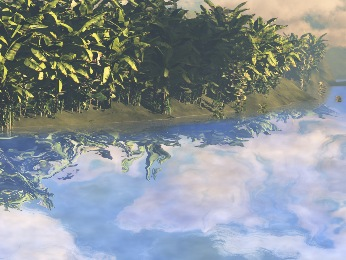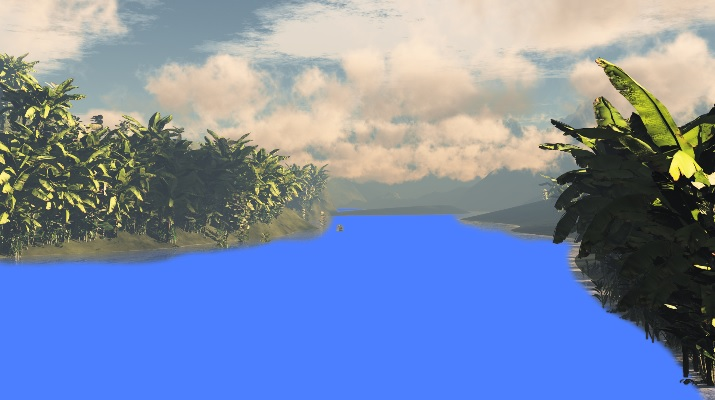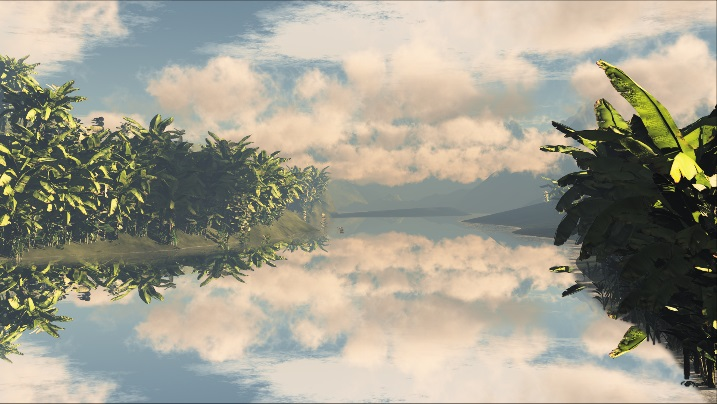Dynamic Water Reflections In Prerendered Scenes
Posted on August 6th, 2016 by Gio
Do you have a background image with some water? Well, the water doesn't need to be static: with literally 10 lines of shader code you can make it look so much more interesting.

Reflections are a fairly complex thing, and you would think that you can only calculate 3D reflections in a 3D scene; once the scene has been rendered into a 2D picture, there isn't much you can do to add dynamic reflections, right? Wrong of course! There are lots of tricks you can use to achieve good looking (if not quite physically accurate) reflections.

Today I'm going to show you how to do something like this thing below with just a bunch of shader instructions. You can also switch to full screen and see this simple effect in glorious full-HD.

Obviously we need a pre-rendered picture to start with. Here I've quickly created one using an excellent program called VUE, and saved it as a .png file. I have then edited the alpha channel of this file to make it transparent in all the places where the water would be. I ended up with this:This is the same old trick that we've used a few times before: use the alpha channel as a mask, to mark the areas of the image where we want to apply a specific effect.

I then opened WADE, imported the picture and created a sprite that uses it. I assigned this simple shader to it (when doing this, remember to click the Always Draw button in the sprite's properties):

```vec4 color = texture2D(uDiffuseSampler, uvAlphaTime.xy);
vec4 waterColor = vec4(0.3,0.5,1.,1.);
color = mix(waterColor, color, color.w);
color.w = 1.;
gl_FragColor = color;
```

It's pretty simple really: we sample our diffuse texture as usual, and also define a blue-ish water color. We then decide wether to display the texture color or the blue-ish color based on the texture color's alpha. This is done using the mix instruction in GLSL, and I'm then setting the alpha explicitly to 1 to ensure that the resulting image is fully opaque.It goes without saying that if you spend a bit more time creating your source image and tweaking the alpha mask, you can get much better results. I've done this pretty quickly, but for the purpose of this blog post it will suffice.

Now if we change our waterColor variable, that's only going to affect the blue part of the picture (the water part, I should say), without touching the rest of it, which is what we want.

The next thing to do is to decide which pixels we actually want to reflect. In reality this is a fairly complex problem: we would need to know what camera matrix was used to project the 3D world onto the 2D plane of the screen to calculate this accurately. Luckily, we don't need accuracy. We just need something that looks good enough, so we're going to make a big assumption: the vector that goes from our eye into the screen is roughly parallel to the water plane. And if that is true, that means that we can approximately mirror the picture, and use the mirrored image as a basis for our reflections.

Because of this big assumption, the code to do this is really simple:

```	waterColor = texture2D(uDiffuseSampler, vec2(uvAlphaTime.x, 1.-uvAlphaTime.y));
```

This reflects the top part of the screen perfectly onto the bottom part. However, because we are going to be adding distortions soon, we want to tweak these numbers slightly, so we don't end up sampling points that are outside our texture. For this very reason, we are going to scale up this reflected image along the X axis, and to move it a bit along Y:

```	waterColor = texture2D(uDiffuseSampler, vec2(((uvAlphaTime.x * 2. - 1.) * 0.95 +1.) / 2., 1.035-uvAlphaTime.y));
```

Note that our uvCoordinates are in the range [0, 1]. To ensure that we scale everything around the center on the X axis, we are multiplying the X coordinate by 2 and subtracting one, therefore moving it to the range [-1, 1]. We can then multiply by a scale factor (0.95), and invert the previous transormation, i.e. we add 1 then divide by 2. This is what we get:It looks nice, but it's still static, and static images are boring! To make it more interesting we are going to add some perturbations to the water. As we've done in previous posts, I'm going to use some Perlin Noise to do this. To be precise, I'll need 3 layers of Perlin noise, that you can create in code or just through GIMP, and combine into a single texture using the R, G and B channels. The important thing is that you make it tileable. This is the texture that I used for the demo above:It's just 3 layers of noise: R and G use a low-frequency noise that we can use to offset our texture sampling, B contains higher-frequency noise that we will overlay on top of everything to give the impression that the water is somewhat flowing.

Let's start by sampling the noise textures, and creating a vector that contains the R and G channels (also known as X and Y). When sampling the noise texture, we add time (uvAlphaTime.w) to the Y coordinates, to give the impression that the noise (and so the water) is flowing downwards. We are going to rescale the range like we did before, so these coordinates we multiply by 2 and subtract 1 to end up in the range [-1, 1].

```	vec4 n = texture2D(noise, uvAlphaTime.xy * 2. - vec2(0., uvAlphaTime.w * 0.01));
vec2 vnoise = (n.xy * 2. - 1.) * 0.15;
```

As you can see I'm also multiplying the resulting vector by 0.15, an arbitrarily small number to control the amount of noise that we are going to add to our reflections. Of course you can choose a different number, the point being that we don't want too much noise, nor too little, and at the end of the day it's just personal preference how big you want this number to be.

Now we've calculated a noise vector, obviously we want to use it! The trick is to just add it to the texture coordinates that we are using to sample our (reflected) water color:

```	vec4 waterColor = texture2D(uDiffuseSampler, vec2(((uvAlphaTime.x * 2. - 1.) * 0.95 +1.) / 2., 1.035-uvAlphaTime.y) + vnoise);
```

While that may work, it could be better, because we are not taking perspective into account. Pixels that are farther away from us (so higher up on the screen) should be receiving less noise. This is easily done: we can just scale our noise vector (vnoise) based on the vertical texture coordinate (uvAlphaTIme.y). Because we only have water on the bottom half of the screen, I'm going to subtract 0.5 from the vertical texture coordinate, so that the point on the middle of the screen will receive no noise, and points closer to the bottom will receive more and more noise:

```	vnoise *= max(0., (uvAlphaTime.y - 0.5) * 2.);
```

The effect is now pretty much complete, but I'd like to add a couple of finishing touches. Rather than just using our reflections for the water color, I think it looks better if we mix the reflections with some solid blue-ish color. Instead of using a constant factor for the mixing, I'm going to use a channel of the noise texture (the green channel, but I could have used any other), so we get a non-uniform mix of reflections and a solid color.

```	waterColor = mix(waterColor, vec4(0.3,0.5,1.,1.), n.y * 0.6);
```

The 0.6 at the end is just an arbitrary factor to indicate that I always want some reflections, i.e. the solid blue color should never be more than 60% of the total result.

Finally, I want to add some monochrome noise on top of everything, to make the water surface appear less regular. This is where the high frequency noise (the blue channel of our noise texture) can be useful. I will sample it adding time to the Y texture coordinate, so it appears to scroll downwards, giving the impression of a flowing liquid. I want this bit to be very subtle, so I'm multiplying the effect for a small factor, 0.15 in this case.

```	waterColor.xyz += texture2D(noise, uvAlphaTime.xy * 2. - vec2(0., uvAlphaTime.w * 0.02)).zzz * 0.15;
```

```	vec4 color = texture2D(uDiffuseSampler, uvAlphaTime.xy);
vec4 n = texture2D(noise, uvAlphaTime.xy * 2. - vec2(0., uvAlphaTime.w * 0.01));
vec2 vnoise = (n.xy * 2. - 1.) * 0.15;
vnoise *= max(0., (uvAlphaTime.y - 0.5) * 2.);
vec4 waterColor = texture2D(uDiffuseSampler, vec2(((uvAlphaTime.x * 2. - 1.) * 0.95 +1.) / 2., 1.035-uvAlphaTime.y) + vnoise);
waterColor = mix(waterColor, vec4(0.3,0.5,1.,1.), n.y * 0.6);
waterColor.xyz += texture2D(noise, uvAlphaTime.xy * 2. - vec2(0., uvAlphaTime.w * 0.02)).zzz * 0.15;
color = mix(waterColor, color, color.w);
color.w = 1.;
gl_FragColor = color;
```

If you want you can download the complete WADE project, and play with the values a bit. Enjoy!